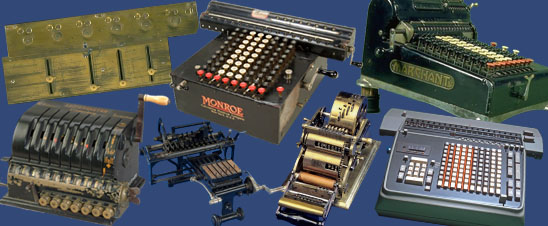# Calculating Machines -- Difference Engines

## Difference EnginesCalculating machines were sometimes used to compute and print the mathematical tables widely used by nineteenth century engineers, mathematicians and scientists. A small number of digital computing machines computed and printed mathematical tables by finding successive values of a function using a mathematical technique known as the method of finite differences. The Englishman Charles Babbage proposed such a “difference engine” in 1820, and built a small section of it. Difference engines actually were built by Georg and Edvard Scheutz in Sweden and by George B. Grant in the United States. These pioneering devices were not widely adopted.

## Contents